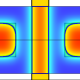## Prefix-free codes and ordinals

Very nice. I like that the ordinal based construction allows for quite some freedom, while still ensuring that every number can be represented uniquely, and that lexicographically greater codewords map to bigger numbers.

Allowing non-binary digits would provide even more freedom. I guess the only thing which would change in the ordinal based construction is that (2n-1+b) would be replaced by (rn-r+1+d), where d is an r-ary digit. And the encoding of$1^n0x$ would have to be adapted too.

I wonder what motivated you to write this post. Was it just the obvious motivation to construct a connection between ordinals and natural number representations?What Immanuel Kant teach you

Consider the problem of representing a number in computer memory, which is idealized as a sequence of zeros and ones. The binary number system is a well-known solution to this problem — for example, the sequence “01101” represents \$latex 0 cdot 2^4 + 1 cdot 2^3 + 1 cdot 2^2 + 0 cdot 2^1 + 1 cdot 2^0 = 11\$. But there’s a problem: You don’t expect the entire computer to just be used to represent one number; you expect it to have other things stored afterwards. So how do you tell where the number ends? If the sequence begins \$latex 01101001dots\$ does this represent the number \$latex 011_2\$ or \$latex 01101_2\$ or \$latex 0110100_2\$?

The solution to this problem most commonly used in practice is to declare in advance a fixed number of bits that will be used to represent the number, usually 32 bits or 64 bits. For…

View original post 1,814 more words